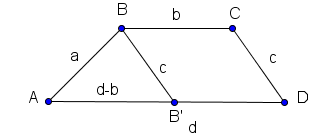# Solve a Trapezoid Given its Bases and Legs

A trapezoid with bases b and d (d > b), legs a and c, and AD and BC are parallel is shown below . Calculate all its angles and its height h.## Angles of a Trapezoid

Using the trapezoid above, we draw BB' parallel to CD.Using the triangle ABB', we use the cosine rule to write
c
2 = a2 + (d-b)2 - 2 a (d - b) cos(∠BAD)
2 + (d-b)2 - c2) / (2 a (d - b) )
∠BAD = arccos ( (a2 + (d-b)2 - c2) / (2 a (d - b) ) )

In the same figure, ∠BB'A and ∠CDA have the same size.
Using the same triangle, we use the cosine rule again to write
a
2 = c2 + (d-b)2 - 2 c (d - b) cos(∠BB'A)
cos(∠BB'A) = (c
2 + (d-b)2 - a2) / (2 c (d - b) )
∠CDA = ∠BB'A = arccos ( (c2 + (d-b)2 - a2) / (2 c (d - b) ) )

In the given trapezoid, AD and BC are parallel. Hence the pairs of angles BAD and ABC and CDA and DCB are supplementary. Hence
ABC = 180� - BAD and DCB = 180� - CDA

## Height and Area of a Trapezoidarea = (1/2)(b + d) h

## Diagonals of a TrapezoidUse cosine rule in triangles DAB and BCD to write:
BD
2 = a2 + d2 - 2 a d cos (∠ BAD)
BD = √ (a2 + d2 - 2 a d cos (∠ BAD))

CA
2 = c2 + d2 - 2 c d cos (∠ CDA)
CA = √ (c2 + d2 - 2 c d cos (∠ CDA))

## More References and Links to Geometry

Geometry Tutorials, Problems and Interactive Applets.
Trapezoid Area Calculator. Calculator to calculate the area of a trapezoid given the bases and the height.
Trapezoid Calculator and Solver. An easy to use online calculator to solve trapezoid problems. The area, the angles and the diagonals of a Trapezoid are calculated given its 4 sides.